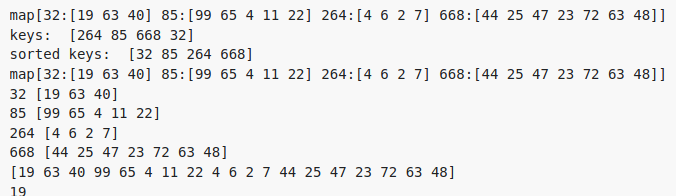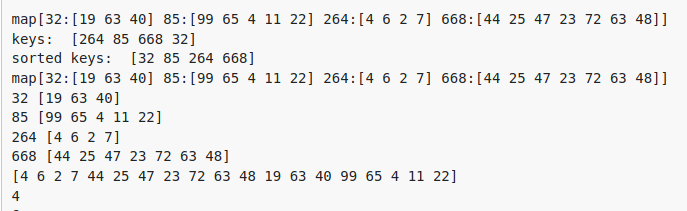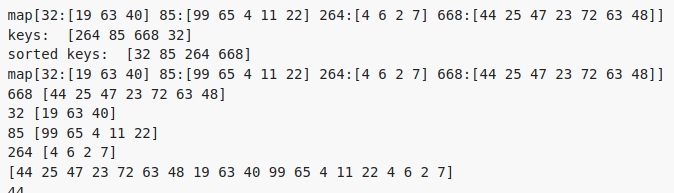# Sorted maps and slices, getting a different result for each compilation

Hello everyone,

Could someone help me understand what’s going on with the following code, I don’t know why it gave me different results each time I ran it. My goal is to create a map and sort it by keys, and then I want to gather its values in a slice.

package main

import (
“fmt”
“sort”
)

func main() {
map1 := make(map[uint16][]byte)
map1 = []byte{4, 6, 2, 7}
map1 = []byte{99, 65, 4, 11, 22}
map1 = []byte{44, 25, 47, 23, 72, 63, 48}
map1 = []byte{19, 63, 40}
fmt.Println(map1)
keys := make([]uint16, 0)
for key := range map1 {
keys = append(keys, key)
}
fmt.Println("keys: ", keys)
sort.Slice(keys, func(i, j int) bool { return keys[i] < keys[j] })
fmt.Println("sorted keys: ", keys)
sort_map := make(map[uint16][]byte)
for k := range keys {
sort_map[keys[k]] = map1[keys[k]]
}
fmt.Println(sort_map)
for k, v := range sort_map {
fmt.Println(k, v)
}
total_data := make([]byte, 0)
for _, v := range sort_map {
total_data = append(total_data, v…)
}
fmt.Println(total_data)
for i := range total_data {
x := total_data[i]
fmt.Println(x)
}
}

Thank you so much for your help, I really appreciate it.

Maps are unsortable. You can instead sort a slice of keys like you are already doing and then just iterate over that slice whenever you need the data in order.

1 Like

Thank you so much for your quick reply. I already did that but I don’t know why it gave different results each time I compile it.

This one is correctThese two results are not.I’m not sure I understand what you’re trying to do, but I removed `sort_map` and came up with this. Is this what you’re looking for?

1 Like

Yes, thank you so much for your help